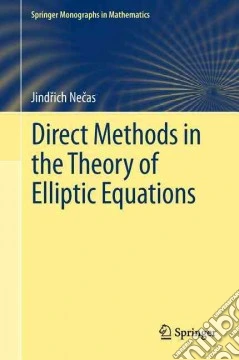ricerca
avanzata

# Direct Methods in the Theory of Elliptic Equations

## Un libro in lingua di Jindrich NecasNecasova Sarka (EDT)Simader Christian G. (CON) edito da Springer Verlag, 2011

• € 95,30
• Il prezzo è variabile in funzione del cambio della valuta d’origineNecas' book Direct Methods in the Theory of Elliptic Equations, published 1967 in French, has become a standard reference for the mathematical theory of linear elliptic equations and systems. This English edition, translated by G. Tronel and A. Kufner, presents Necas' work essentially in the form it was published in 1967. It gives a timeless and in some sense definitive treatment of a number issues in variational methods for elliptic systems and higher order equations. The text is recommended to graduate students of partial differential equations, postdoctoral associates in Analysis, and scientists working with linear elliptic systems. In fact, any researcher using the theory of elliptic systems will benefit from having the book in his library.The volume gives a self-contained presentation of the elliptic theory based on the "direct method", also known as the variational method. Due to its universality and close connections to numerical approximations, the variational method has become one of the most important approaches to the elliptic theory. The method does not rely on the maximum principle or other special properties of the scalar second order elliptic equations, and it is ideally suited for handling systems of equations of arbitrary order. The prototypical examples of equations covered by the theory are, in addition to the standard Laplace equation, Lame's system of linear elasticity and the biharmonic equation (both with variable coefficients, of course). General ellipticity conditions are discussed and most of the natural boundary condition is covered. The necessary foundations of the function space theory are explained along the way, in an arguably optimal manner. The standard boundary regularity requirement on the domains is the Lipschitz continuity of the boundary, which "when going beyond the scalar equations of second order" turns out to be a very natural class. These choices reflect the author's opinion that the Lame system and the biharmonic equations are just as important as the Laplace equation, and that the class of the domains with the Lipschitz continuous boundary (as opposed to smooth domains) is the most natural class of domains to consider in connection with these equations and their applications.